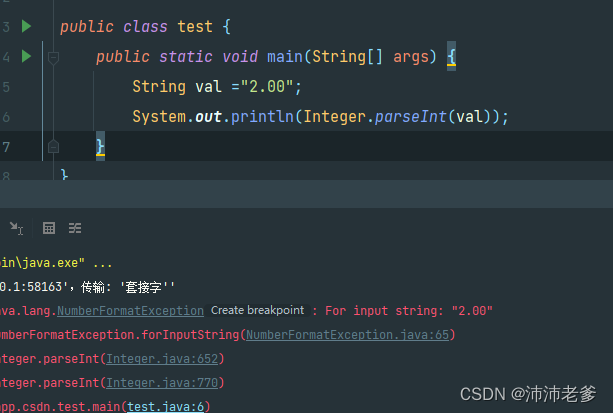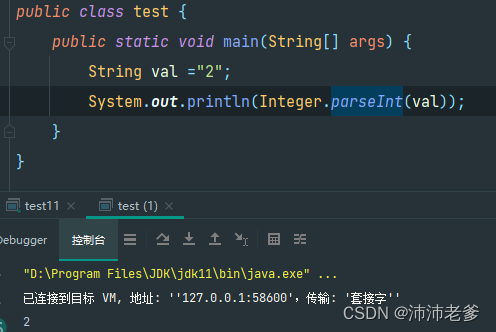# [Solved] Java Run Error: For input string: “XXX”

Background

Today, a small partner of front-end joint commissioning said that an interface reported an error.

I first glanced at the code and found no problem.

First post the code of the place where the error is reported

``````...
IntegralRecord integralRecord = new IntegralRecord();
integralRecord.setUid(uid);
integralRecord.setIntegral(Integer.parseInt(amount));
integralRecord.setStatus(1);
integralRecord.setMark(StrUtil.format("Free {} points for completing tasks", amount));
userIntegralRecordService.save(integralRecord);
...``````

Problem location
There is no choice but to debugger a line to run.

After running to

integralRecord.setIntegral(Integer.parseInt(amount));

This line, then reported an error message For input string.

This line of code, when I look at it, just does a conversion operation on the type.

Is there something wrong with the passed data?

I ran it again and found that amount=2.00

Explanation: Because the amount is shared by several places, considering that there may be a decimal situation, a String is used in the front-end pass, and the back-end uses the corresponding type for conversion according to the actual situation.

When converting, it is indeed this line that has a problem.

The specific test situation is shown below:Then we changed it to

amount=”2″

The results are as followsThen the problem can be defined because integer cannot recognize the case with decimals during conversion.Integer.parseInt source code

We found that parseInt finally called the following code.

``````public static int parseInt(String s, int radix) throws NumberFormatException{

if (s == null) {
throw new NumberFormatException("null");
}

}

}

boolean negative = false;
int i = 0, len = s.length();
int limit = -Integer.MAX_VALUE;

if (len > 0) {
char firstChar = s.charAt(0);
if (firstChar < '0') { // Possible leading "+" or "-"
if (firstChar == '-') {
negative = true;
limit = Integer.MIN_VALUE;
} else if (firstChar != '+') {
throw NumberFormatException.forInputString(s);
}

if (len == 1) { // Cannot have lone "+" or "-"
throw NumberFormatException.forInputString(s);
}
i++;
}
int result = 0;
while (i < len) {
if (digit < 0 || result < multmin) {
throw NumberFormatException.forInputString(s);
}
if (result < limit + digit) {
throw NumberFormatException.forInputString(s);
}
result -= digit;
}
return negative ?result : -result;
} else {
throw NumberFormatException.forInputString(s);
}
}``````

Source code positioning

In the code, we can see that an error will be reported if the range is no longer specified

``````int digit = Character.digit(s.charAt(i++), radix);
if (digit < 0 || result < multmin) {
throw NumberFormatException.forInputString(s);
}``````

java.lang.Character.digit(char ch, int radix) method :

Returns the value of the character ch in the specified base, if the base is not in the range MIN_RADIX ≤ base ≤ MAX_RADIX or if the value of the channel is not a valid number in the specified base -1, then it is returned.

The value of radix is 10, this is because the conversion Int type is when the single character 0~9.

So if the value is not a number between 0~9 an error will be reported.

The exception thrown is forInputString.Solution:
String interception
In this example, it is clear that only the integer part is needed, so you can specify the character “.” based on the to intercept.

Then you can convert it and it’s done.

Borrowing from a third party
Here we recommend using the NumberUtil from the hutool toolkit

You can convert similar numbers directly.

Method: NumberUtil.parseInt

`````` String val ="2.00";
int i = NumberUtil.parseInt(val);``````

Type conversion

Because it can be determined that the value must be of numerical type.

So it can be directly converted to BigDecimal type.

BigDecimal has an intValue method, which can be called after direct conversion

`````` String val ="2.00";
int i = new BigDecimal(val).intValue();``````